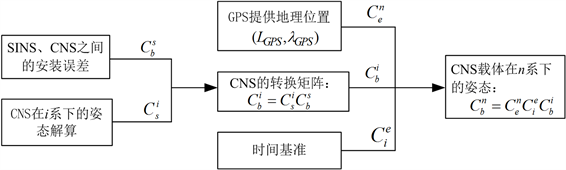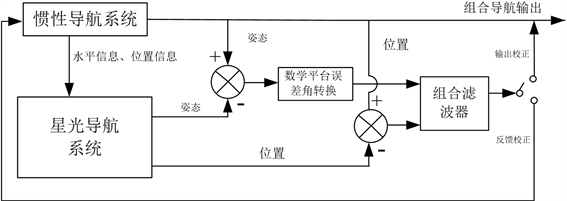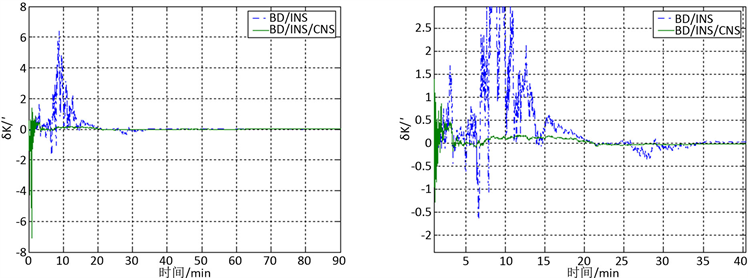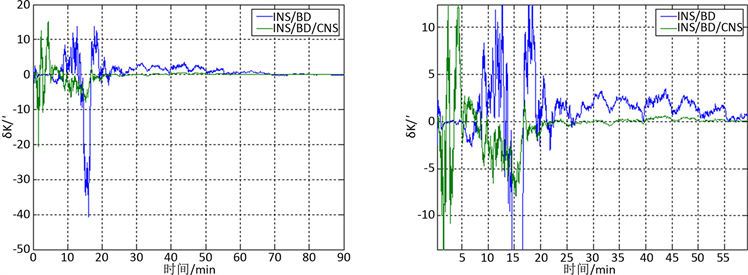﻿ 惯性/星光组合导航系统动基座对准应用

# 惯性/星光组合导航系统动基座对准应用INS/CNS Integrated Navigation System Dynamic Alignment Application

Abstract: Aiming at the problem of the alignment of the dynamic base of the INS/CNS integrated navigation system, a method of dynamic base alignment based on the inertial navigation error comprehensive calibration mode is designed. Based on the attitude and position measurement information of the INS/CNS combination system, the linearized state equation of the INS/CNS combination system is derived, and the measurement equation of the alignment mode is analyzed and established. The Kalman filter technology is used to design the information based on this information. The dynamic base alignment scheme of the fusion method is verified by simulation analysis. The simulation re-sults show that the method is effective under dynamic base alignment. The simulation results show that the method can achieve better than 15’’ within 60 minutes under the dynamic navigation conditions of the ship heading accuracy.

1. 引言

2. 组合系统动基座对准

3. 动基座下量测信息转换

${C}_{b}^{n}={C}_{e}^{n}{C}_{i}^{e}{C}_{s}^{i}{C}_{b}^{s}$ (1)Figure 1. CNS information transformation based on GPS assistance

4. 组合系统滤波模型设计

1) 建立惯导状态方程

${X}_{13×1}={\left[{\varphi }_{x},{\varphi }_{y},{\varphi }_{z},\delta {v}_{x},\delta {v}_{y},\delta L,\delta \lambda ,{\epsilon }_{x},{\epsilon }_{y},{\epsilon }_{z},{\nabla }_{x},{\nabla }_{y},{\nabla }_{z}\right]}^{\text{T}}$Figure 2. Principle of INS/CNS attitude + position combination mode

2) 建立天文/惯导组合导航系统量测方程

$\left\{\begin{array}{l}\delta \gamma =-\frac{1}{\mathrm{cos}\theta }\left({\varphi }_{x}\mathrm{sin}\psi +{\varphi }_{y}\mathrm{cos}\psi \right)\\ \delta \theta =-{\varphi }_{x}\mathrm{cos}\psi +{\varphi }_{y}\mathrm{sin}\psi \\ \delta \psi =-\frac{1}{\mathrm{cos}\theta }\left({\varphi }_{x}\mathrm{sin}\psi \mathrm{sin}\theta +{\varphi }_{y}\mathrm{cos}\psi \mathrm{sin}\theta -{\varphi }_{z}\mathrm{cos}\theta \right)\end{array}$ (2)

$\left[\begin{array}{c}\delta \gamma \\ \delta \theta \\ \delta \psi \end{array}\right]={H}_{\varphi }\left[\begin{array}{c}{\varphi }_{x}\\ {\varphi }_{y}\\ {\varphi }_{z}\end{array}\right]$ (3)

${H}_{\varphi }=-\frac{1}{\mathrm{cos}\theta }\left[\begin{array}{ccc}\mathrm{sin}\psi & \mathrm{cos}\psi & 0\\ \mathrm{cos}\psi \mathrm{cos}\theta & -\mathrm{sin}\psi \mathrm{cos}\theta & 0\\ \mathrm{sin}\psi \mathrm{sin}\theta & \mathrm{cos}\psi \mathrm{sin}\theta & -\mathrm{cos}\theta \end{array}\right]$ (4)

${Z}_{1}\left(t\right)=\left[\begin{array}{c}{\varphi }_{E_INS}-{\varphi }_{E_CNS}\\ {\varphi }_{N_INS}-{\varphi }_{N_CNS}\\ {\varphi }_{U_INS}-{\varphi }_{U_CNS}\end{array}\right]={H}_{1}\left(t\right)X\left(t\right)+V\left(t\right)$ (5)

3)建立卫导/惯导量测

${Z}_{2}\left(t\right)=\left[\begin{array}{c}{\phi }_{INS}-{\phi }_{GNSS}\\ {\lambda }_{INS}-{\lambda }_{GNSS}\end{array}\right]={H}_{2}\left(t\right)X\left(t\right)+V\left(t\right)$ (6)

$Z\left(t\right)=\left[\begin{array}{c}\alpha \\ \beta \\ \gamma \\ \delta \phi \\ \delta \lambda \end{array}\right]=H\left(t\right)X\left(t\right)+V\left(t\right)$ (7)

5. 仿真比较Figure 3. Simulation results under mooring conditionsFigure 4. Simulation results under navigation conditions

6. 结论

 Long, R., Qin, Y.Y. and Xia, J.H. (2011) A New and Effective Algorithm of Attitude Matching for INS/Star Sensor Integrated Navigation System. Journal of North western Polytechnical University, 29, 476-479.

 Bona, B.E. and Smay, R.J. (1966) Optimum Reset of Ship’s Inertial Navigation System. IEEE Transactions on Aerospace and Elec-tronic Systems, 2, 409-414.
https://doi.org/10.1109/TAES.1966.4501790

 Zhou, L.-F., Zhao, X.-M., et al. (2015) Initial Alignment of CNS/INS Integrated Navigation System Based on Recursive Least Square Method. Journal of Chinese Inertial Technology, 23, 357-363.

 Zhou, L.-F., Zhao, X.-M., et al. (2017) CNS/SINS Integrated Cali-bration Technique Based on Damping. Journal of Chinese Inertial Technology, 25, 561-565.

 Gao, W., Ben, Y.Y., Zhang, X., Li, Q. and Yu, F. (2011) Rapid Fine Strapdown INS Alignment Method under Marine Mooring Condition. IEEE Transactions on Aerospace and Electronic Systems, 47, 2887-2896.
https://doi.org/10.1109/TAES.2011.6034671

 Sun, F., Xia, J.Z., Lan, H.Y. and Liu, X.T. (2012) Research on Integrated Alignment of Rotary Strapdown Inertial Navigation System. Proceedings of 2012 IEEE International Con-ference on Mechatronics and Automation, Chengdu, 5-8 August 2012, 725-730.
https://doi.org/10.1109/ICMA.2012.6283232

Top